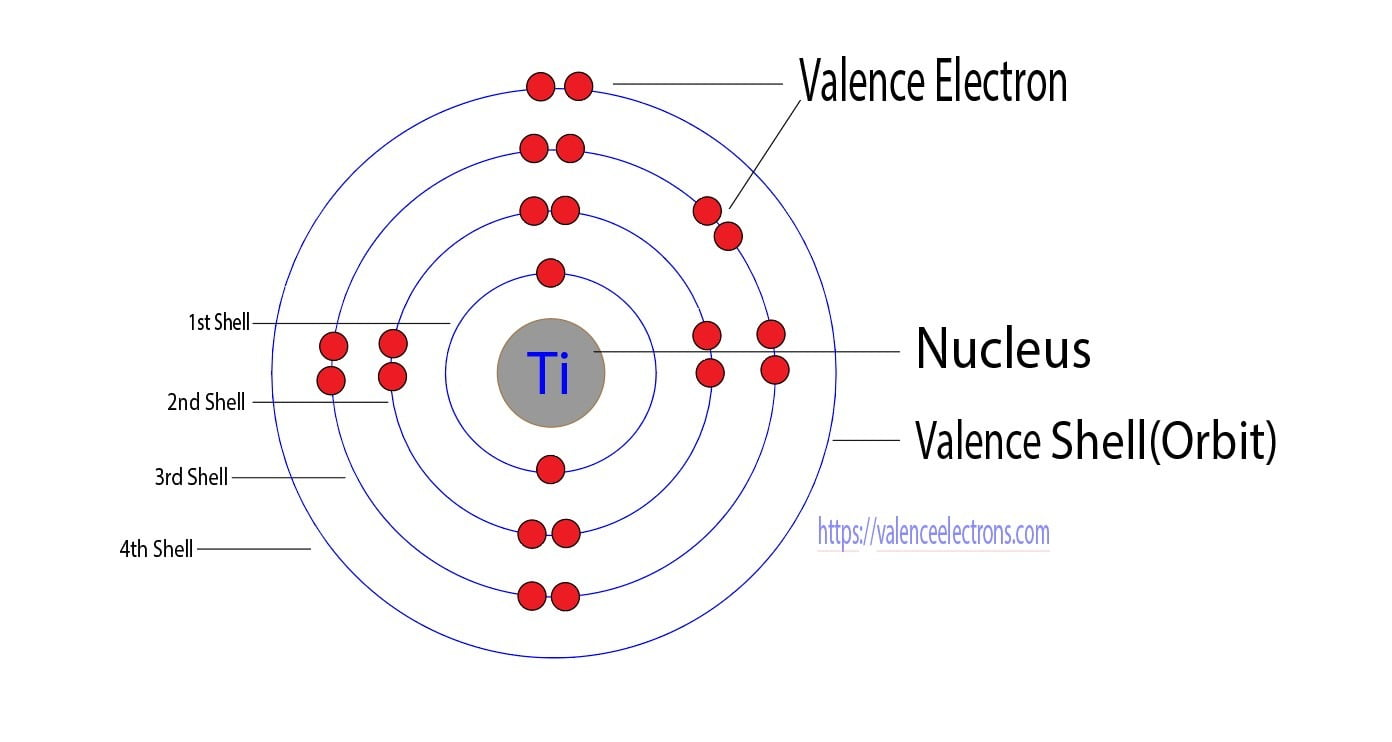## How many valence electrons does titanium(Ti) have?

Titanium is a transition element. Therefore, the valence electrons of titanium are determined differently. The last orbit of a titanium element has two electrons but its valence electrons are not two. This article discusses in detail the valence electrons of titanium. Hopefully, after reading this article you will know in detail about the valence electrons …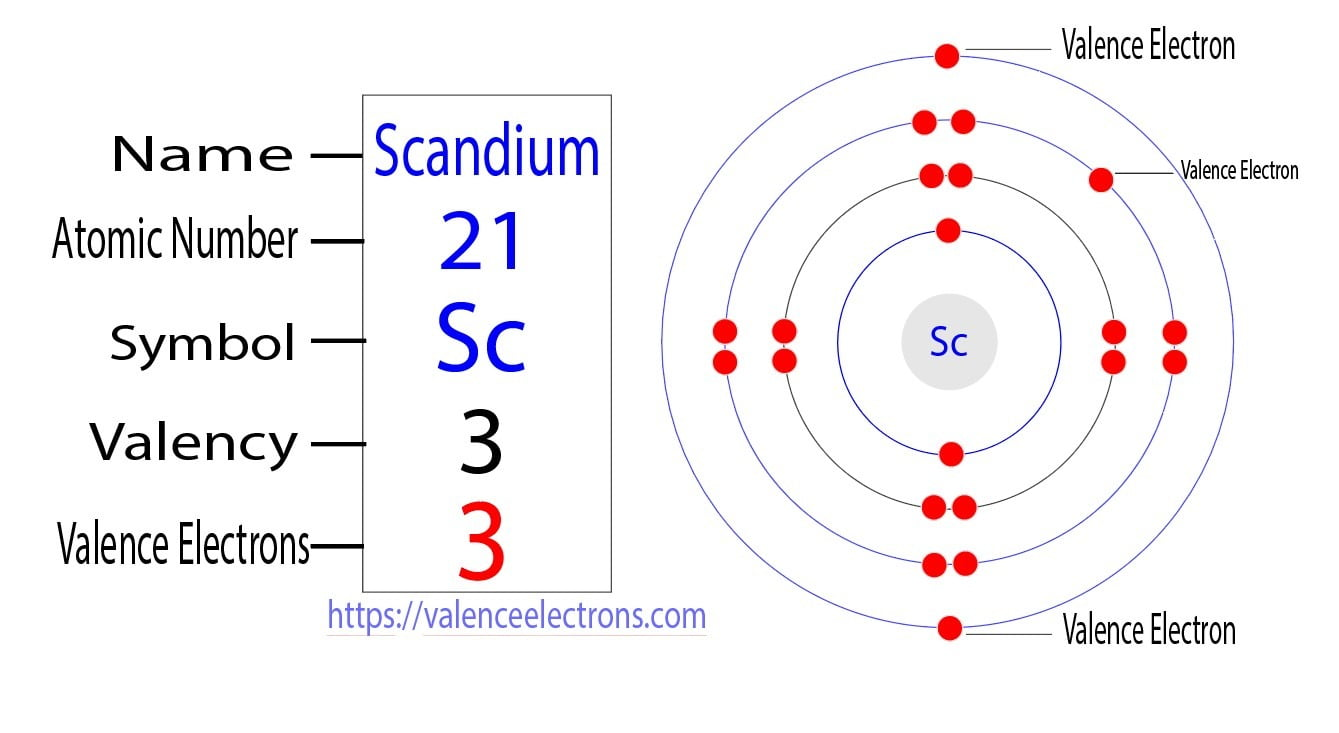## How many valence electrons does scandium(Sc) have?

The 21th element in the periodic table is scandium. The element of group-3 is scandium and its symbol is ‘Sc’. Scandium participates in the formation of bonds through its valence electrons. This article discusses in detail the valence electrons of scandium(Sc). Table of Contents How many electrons and protons does scandium(Sc) have?What are the valence …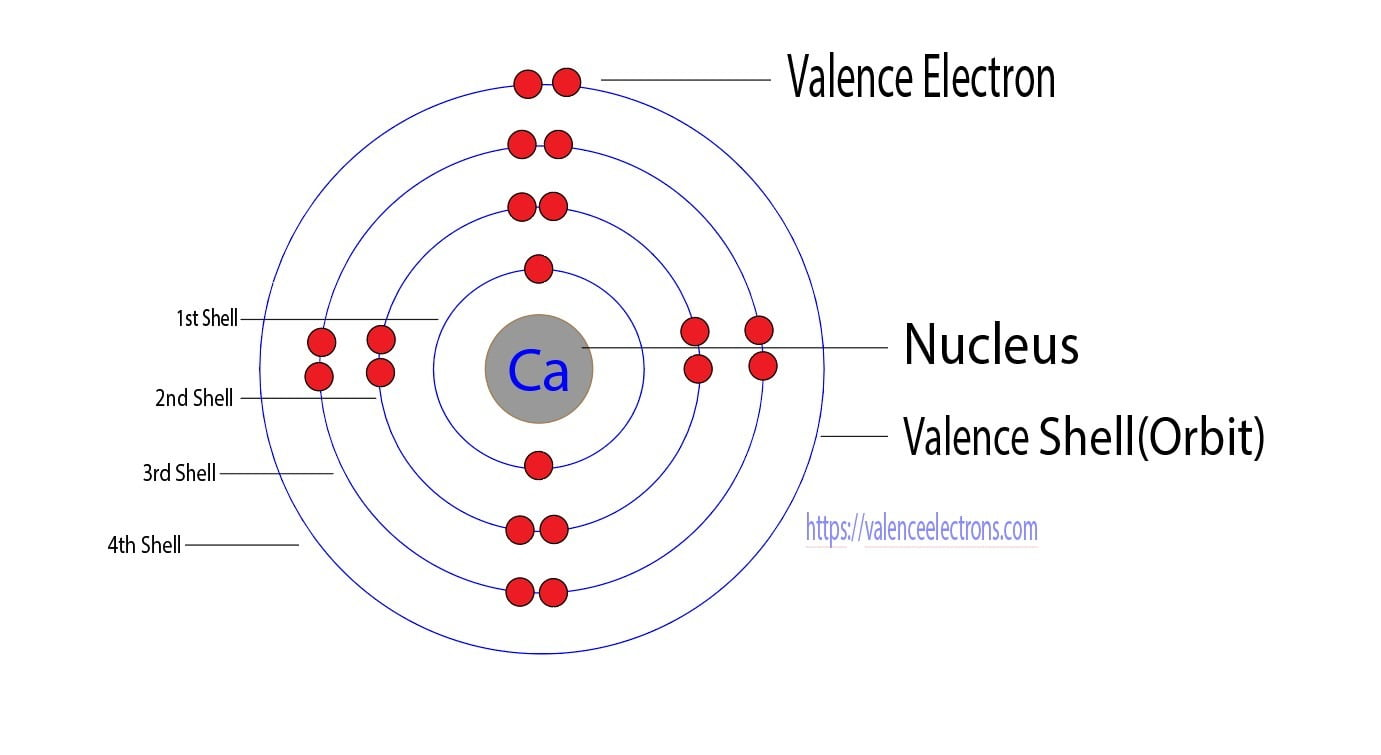## How many valence electrons does calcium(Ca) have?

The 20th element in the periodic table is calcium. Calcium is an alkaline earth metal and its symbol is ‘Ca’. Calcium participates in the formation of bonds through its valence electrons. This article discusses in detail the valence electrons of calcium(Ca). Table of Contents How many electrons and protons does the calcium atom have?What are …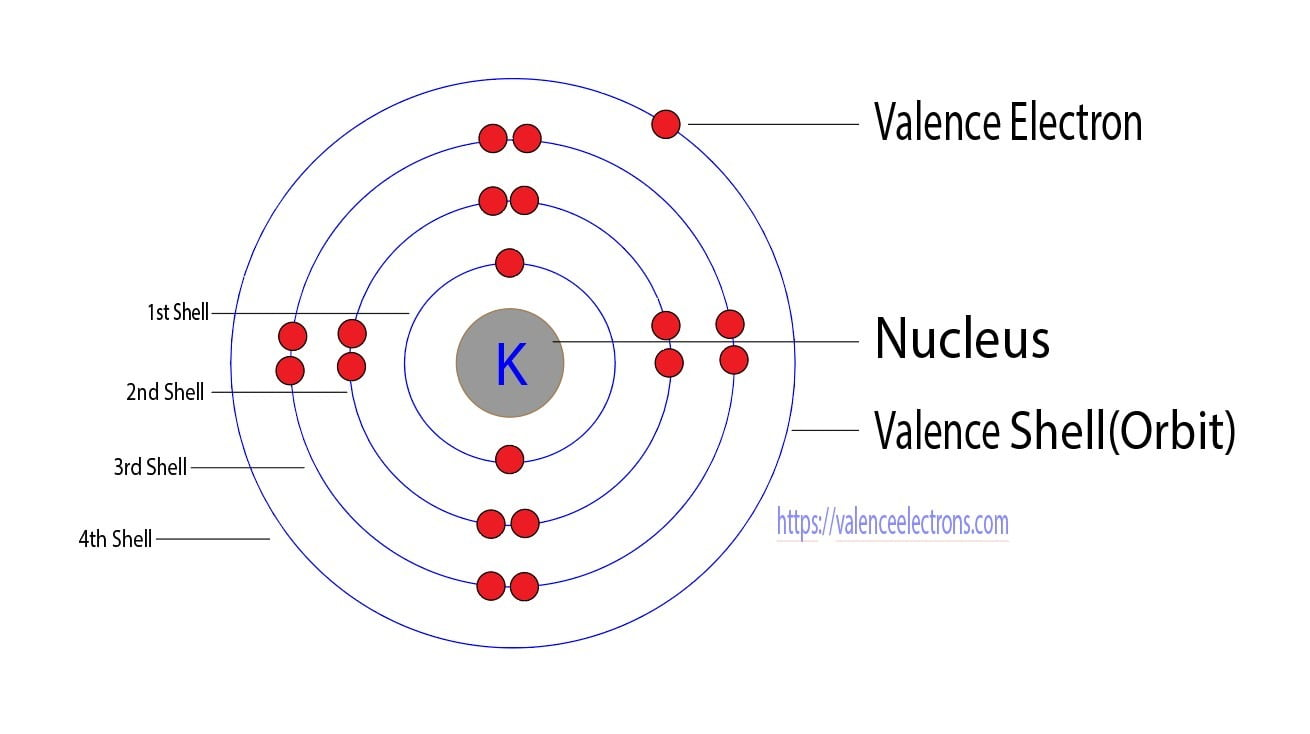## How many valence electrons does potassium(K) have?

Potassium is the 19th element in the periodic table. Potassium is an alkali metal and its symbol is ‘K’. Potassium forms bonds through its valence electrons. This article discusses in detail the valence electron of potassium(K) atom. Table of Contents How many electrons and protons does the potassium(K) atom have?What are the valence electrons of …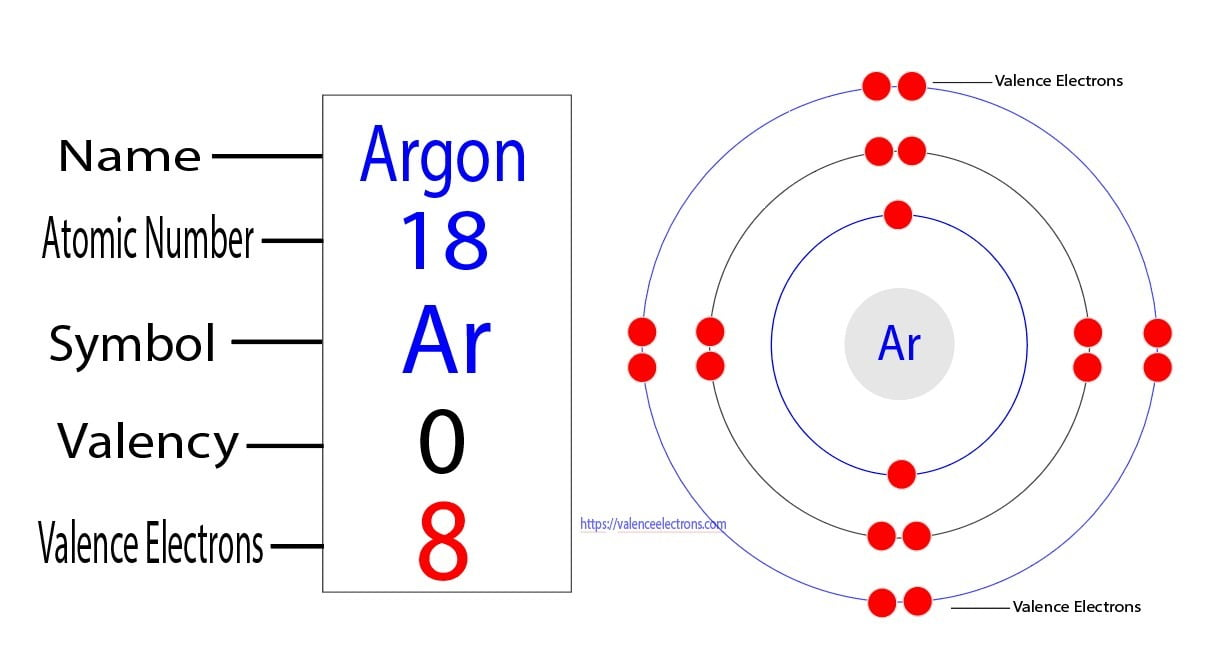## How many valence electrons does argon(Ar) have?

The atomic number of argon is 18. Argon is an inert element and its symbol is ‘Ar’. Argon atoms do not participate in the formation of any bonds. This article discusses in detail the valence electrons of argon(Ar) atom. Table of Contents How many electrons and protons does argon(Ar) have?What are the valence electrons of …## How many valence electrons does chlorine(Cl) have?

The 17th element of the periodic table is chlorine. The element of group-17 is chlorine and its symbol is ‘Cl’. Chlorine forms bonds through its valence electrons. This article discusses in detail the valence electrons of chlorine(Cl). Table of Contents How many electrons and protons does chlorine(Cl) have?What are the valence electrons of chlorine(Cl)?How to …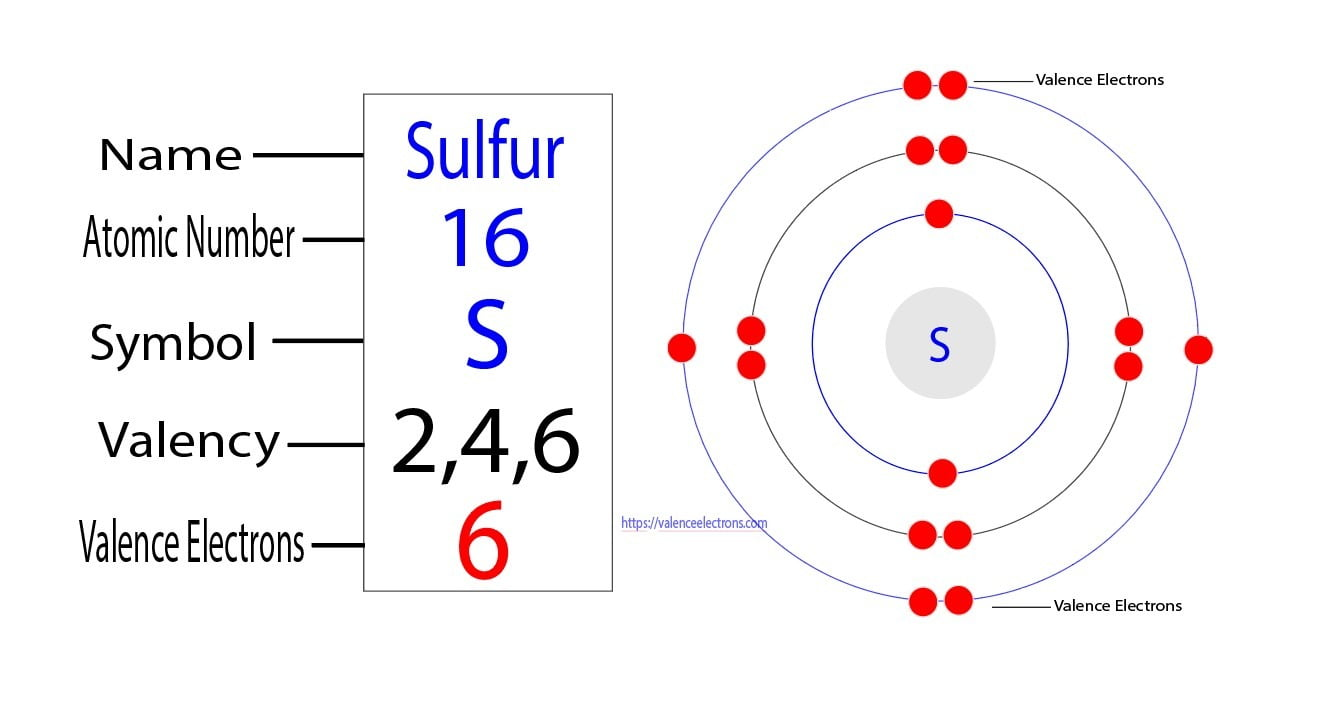## How many valence electrons does sulfur(S) have?

The sixteenth element of the periodic table is sulfur. The element of group-16 is sulfur and, its symbol is ‘S’. Sulfur forms bonds through its valence electrons. This article discusses in detail the valence electrons of sulfur(S). Table of Contents How many electrons and protons does sulfur(S) have?What are the valence electrons of sulfur(S)?How to …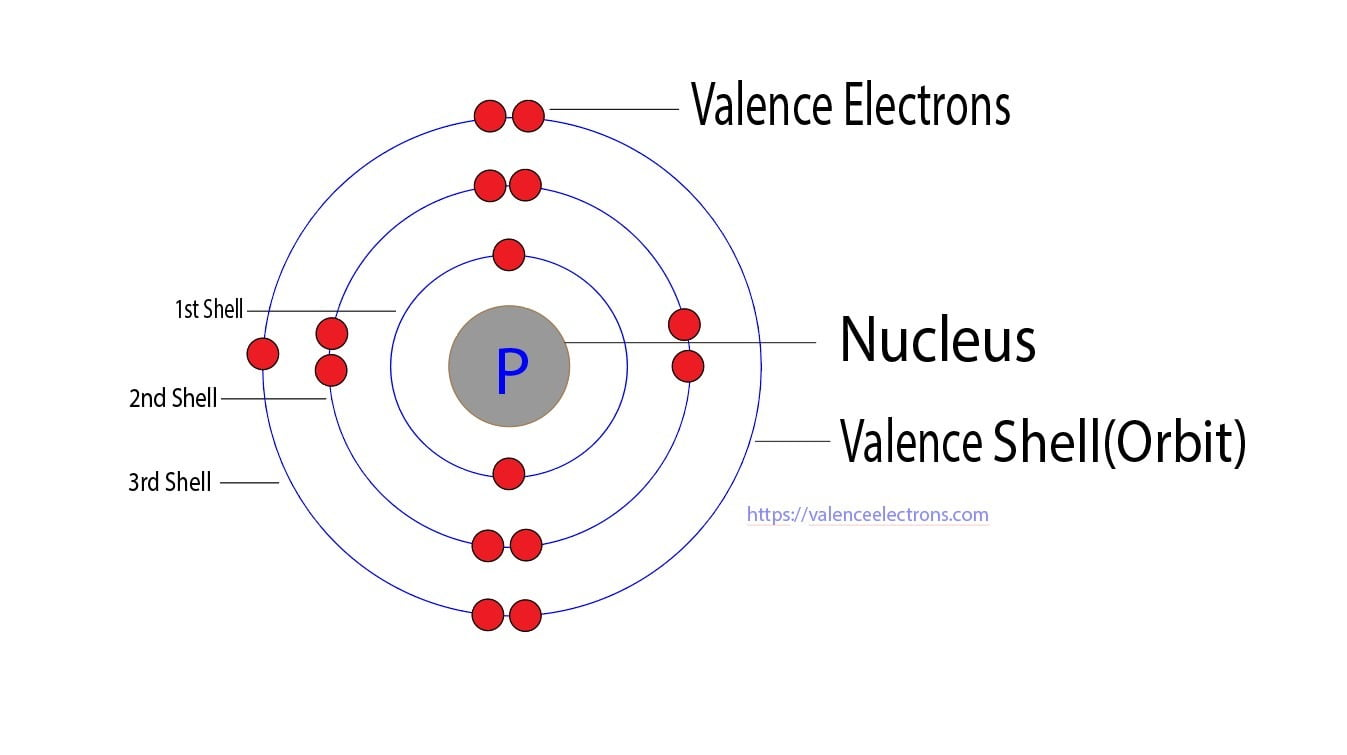## How many valence electrons does phosphorus(P) have?

The fifteenth element of the periodic table is phosphorus. The element of group-15 is phosphorus and, its symbol is ‘P’. Phosphorus forms bonds through its valence electrons. This article discusses in detail the valence electrons of phosphorus(P). Table of Contents How many electrons and protons does phosphorus(P) atom have?What are the valence electrons of phosphorus(P)?How …## How many valence electrons does silicon(Si) have?

The 14th element of the periodic table is silicon. Silicon is a semiconductor material and, its symbol is ‘Si’. Silicon participates in the formation of bonds through its valence electrons. This article discusses in detail the valence electrons of silicon(Si). Table of Contents What are the valence electrons of silicon(Si)?How many electrons and protons does …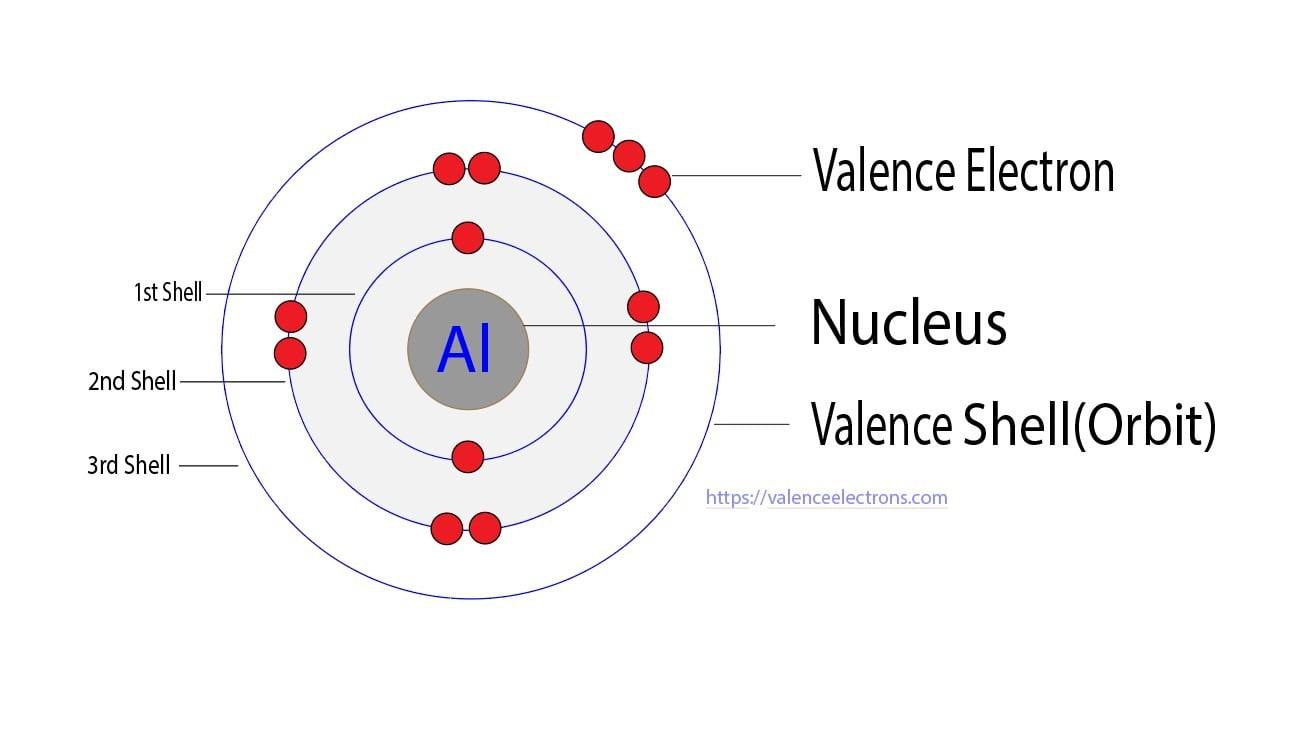## How many valence electrons does aluminum(Al) have?

The thirteenth element of the periodic table is aluminum. The element of group-13 is aluminum and its symbol is Al. Aluminum forms bonds through its valence electrons. This article discusses in detail the valence electrons of aluminum. Table of Contents What are the valence electrons of aluminum(Al)?How many electrons and protons does aluminum(Al) have?How to …